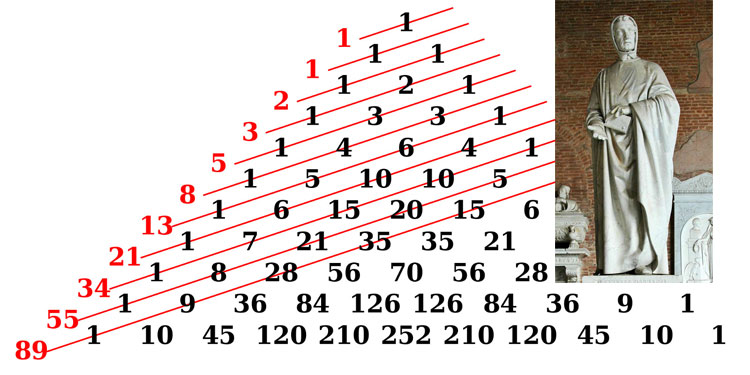A# Fibonacci sequenceThe Fibonacci sequence shown alongside a statue of Fibonacci (Leonardo of Pisa).

The Fibonacci sequence is the sequence that arises in answer to this problem posed in Fibonacci's great work Liber abaci:

A certain man put a pair of rabbits in a place surrounded on all sides by a wall. How many pairs of rabbits can be produced from that pair in a year if it is supposed that every month each pair begins a new pair which from the second month on becomes productive?

The number of pairs of rabbits in the n-th month begins 1, 1, 2, 3, 5, 8, 13, 21, 34, 55, 89, ..., where each term is the sum of the two terms preceding it. This sequence can be defined recursively as follows: F(1) = F(2) = 1, F(n + 1) = F(n) + F(n - 1) for n > 2, where F(n) is the n-th Fibonacci number. Johannes Kepler was the first to point out that the growth rate of the Fibonacci numbers, that is, F(n + 1) / F(n), converges to the golden ratio, φ, which has the value (√5 + 1)/2.

In the 19th century Fibonacci numbers were discovered in many natural forms. For example, many types of flower have a Fibonacci number of petals: daisies tend to have 34 or 55 petals, while sunflowers have 89 or, in some cases, 144. The seeds of sunflowers spiral outward both to the left and the right in a Fibonacci number of spirals. Similarly, the whorls on a pine cone, the numbers of rings on the trunks of palm trees, the patterns of snails shells, and the genealogy of the male bee all follow a sequence of Fibonacci numbers. The arrangement of plant leaves, or phyllotaxis, unfolds to the same pattern because this results in an optimal solution in terms of the spacing of the leaves or the amount of light that can reach them. A familiar spiral form, known as the logarithmic spiral, emerges when seeds on a plant grow and space themselves according the Fibonacci sequence. The logarithmic spiral is approximated by the rule: start at the origin of the Cartesian coordinate system, move F(1) units to the right, move F(2) units up, move F(3) units to the left, move F(4) units down, move F(5) units to the right, and so on. By growing in this way, on structures as such sunflowers, pine cones, and pineapples, seeds are able to pack themselves together most efficiently.

Fibonacci numbers have so many interesting mathematical properties that an entire journal, The Fibonacci Quarterly, is devoted to them. The sequence of final digits in Fibonacci numbers repeats in cycles of 60. The last two digits repeat in 300, the last three in 1500, the last four in 15,000, etc. The product of any four consecutive Fibonacci numbers is the area of a Pythagorean triangle. The shallow (least steep) diagonals of Pascal's triangle sum to Fibonacci numbers. Let m and n be positive integers, then

F(n) divides F(mn)
gcd(F(n), F(m)) = F(gcd(m, n)), where "gcd" stands for "greatest common divisor"
(F(n))2 - F(n+1)F(n-1) = (-1)n-1
F(1) + F(3) + F(5) + ... + F(2n-1) = F(2n)
for every n, there are n consecutive composite Fibonacci numbers.

An interesting use of the Fibonacci sequence is for converting miles to kilometers. For instance, if you want to know about how many kilometers 5 miles is, take the Fibonacci number (5) and look at the next one (8). 5 miles is about 8 kilometers. This works because it happens that the conversion factor between miles and kilometers is roughly equal to the golden ratio.

The first few Fibonacci numbers that are also prime numbers are 3, 5, 13, 89, 233, 1597, 28657, 514229, ... It seems likely that there are infinitely many Fibonacci primes, but this has yet to be proven. However, it is relatively easy to show that for n > or = 4, un+1 is never prime. The Fibonacci sequence is a special case of the Lucas sequence.

The "tribonacci" series is made by adding the last three digits: 1, 1, 2, 4, 7, 13, 24, 44, 81, ... And from this the quadbonacci series, the pentbonacci series, and the hexbonacci series, all the way up to the n-bonacci series. Each ratio of successive terms forms a special constant, analogous to phi.